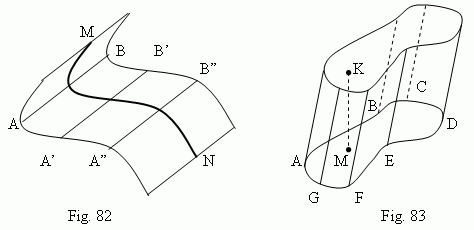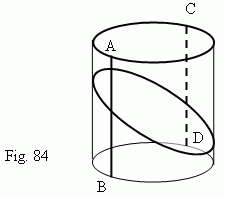# Cylinder

Cylindrical surface. Directrix and generatrices of a cylindrical surface.
Cylinder. Right cylinder. Oblique cylinder. Circular cylinder. Round
cylinder. Prism as a particular case of a cylinder. Cylindrical sections.

Cylindrical surface is a surface, formed by a motion of a straight line (AB, Fig.82 ), which saves the same direction and intersects with the given line MN ( or a curve ). A line MN is called a directrix . Straight lines, corresponding to different positions of the straight line AB at its motion ( A’B’, A”B” etc. ), are called generatrices of cylindrical surface.Cylinder. A body, limited by cylindrical surface (with a closed directrix) and two parallel planes, is called a cylinder ( Fig.83 ). Parts of these planes ( ABCDEFG and abcdefg ) are called bases of a cylinder. A distance between the bases ( KM, Fig.83 ) is a height of a cylinder. A cylinder is right , if its generatrices are perpendicular to its base; otherwise, a cylinder is oblique . A cylinder is circular , if its base is a circle. If a cylinder is simultaneously both right and circular , it is called a round cylinder. A prism is a particular shape of a cylinder ( why ? ).

Cylindrical sections of a circular cylinder lateral surface ( Fig.84 ). The sections, parallel to a base, are circles of the same radius. The sections, parallel to a generatrix of cylinder, are pairs of parallel straight lines (AB || CD). The sections, which are not parallel both to bases and to generatrix, are ellipses .